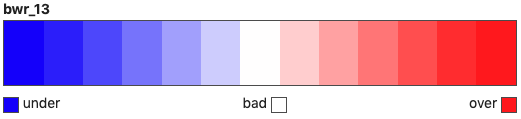# Data visualisation#

## From Data or Index fetchers#

The `argopy.DataFetcher` and `argopy.IndexFetcher` come with a `plot` method to have a quick look to your data. This method can take trajectory, profiler, dac and qc_altimetry as arguments. All details are available in the `argopy.fetchers.ArgoDataFetcher.plot` and `argopy.fetchers.ArgoIndexFetcher.plot` class documentation.

Below we demonstrate major plotting features.

Let’s import the usual suspects:

```from argopy import IndexFetcher as ArgoIndexFetcher
from argopy import DataFetcher as ArgoDataFetcher
```

### Trajectories#

```idx = ArgoIndexFetcher().float([6902745, 6902746]).load()
fig, ax = idx.plot('trajectory')
fig, ax = idx.plot()  # Trajectory is the default plot
```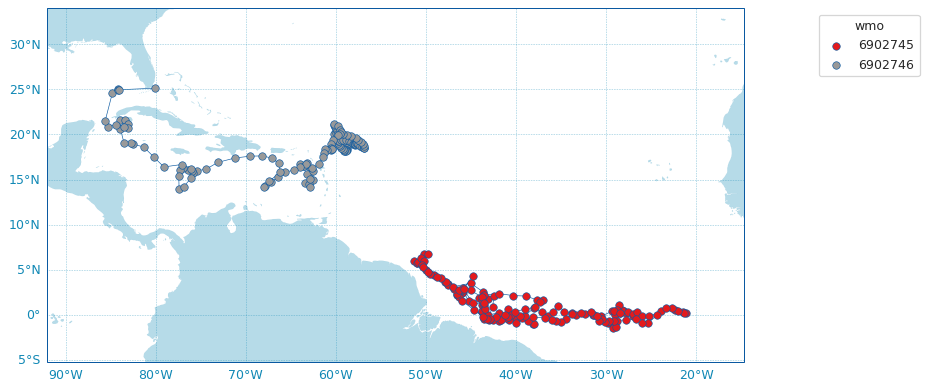### Histograms on properties#

It is also possible to create horizontal bar plots for histograms on some data properties: profiler and dac:

```idx = ArgoIndexFetcher().region([-80,-30,20,50,'2021-01','2021-08']).load()
fig, ax = idx.plot('dac')
```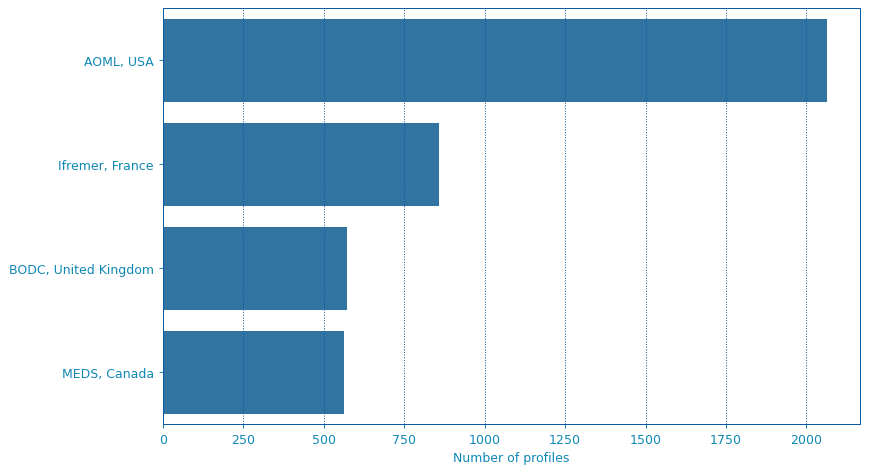If you have Seaborn installed, you can change the plot style:

```fig, ax = idx.plot('profiler', style='whitegrid')
```## Dashboards#

We provide shortcuts to third-party online dashboards that can help you visualise float or profile data. When working in Jupyter notebooks, you can insert a dashboard in a cell, or if you don’t, you can get the url toward the dashboard to open it elsewhere.

We provide access to the Euro-Argo ERIC, Ocean-OPS, Argovis and BGC dashboards with the option `type`. See `argopy.plot.dashboard()` for all the options.

Summary of all available dashboards:

Type name

base

float

profile

“data”, “ea”

X

X

X

“meta”

X

X

X

“bgc”

X

X

X

“ocean-ops”, “op”

X

X

“coriolis”, “cor”

X

“argovis”

X

X

X

Examples:

Open the default dashboard like this:

```argopy.dashboard()
```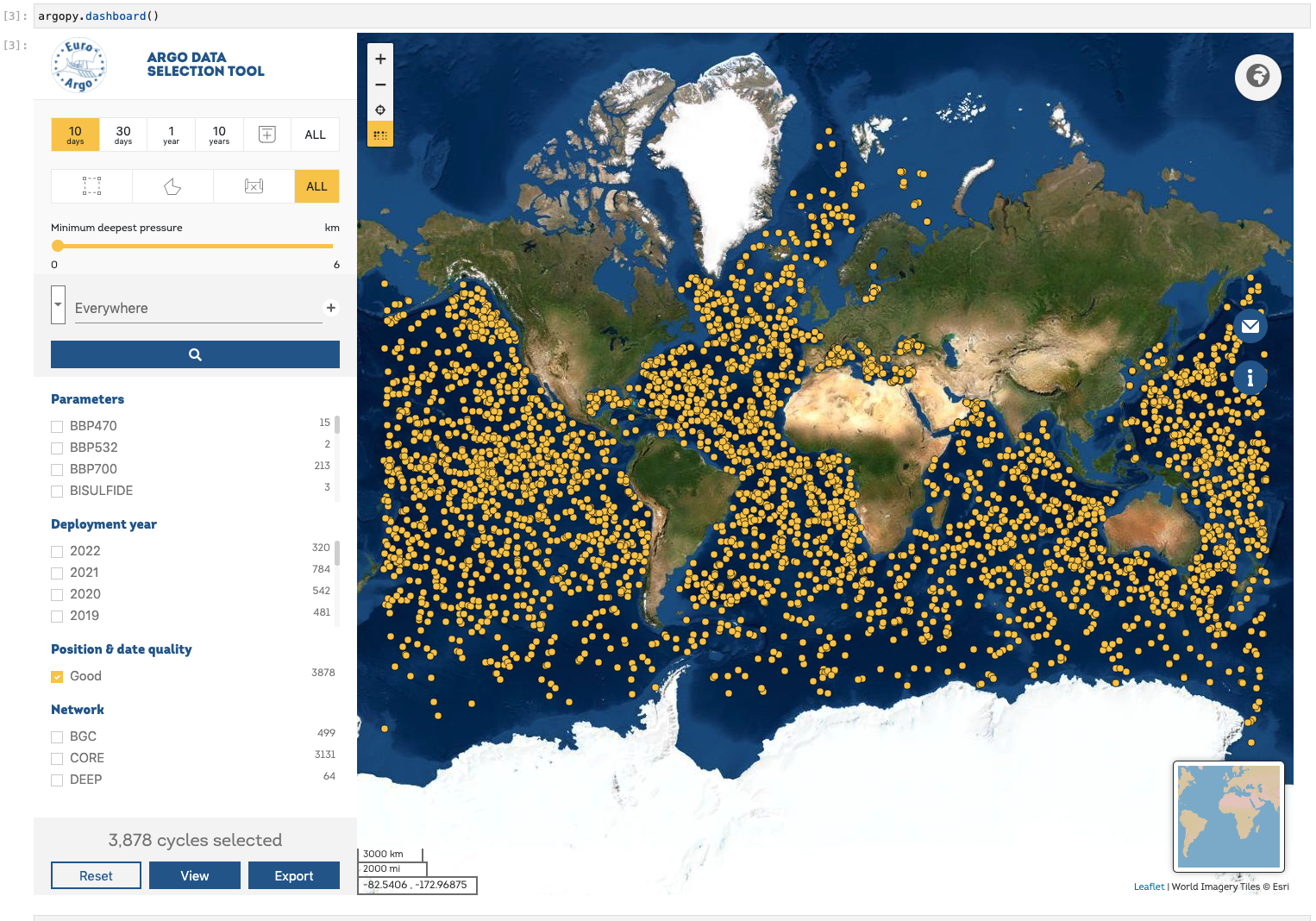Note

Dashboards can be open at the package level or from data fetchers. So that we have the following equivalence:

```argopy.dashboard(WMO)
# similar to:
ArgoDataFetcher().float(WMO).dashboard()
```

and:

```argopy.dashboard(WMO, CYC)
# similar to:
ArgoDataFetcher().profile(WMO, CYC).dashboard()
```

## Scatter Maps#

The `argopy.plot.scatter_map` utility function is dedicated to making maps with Argo profile positions coloured according to specific variables: a scatter map.

Profiles colouring is finely tuned for some variables: QC flags, Data Mode and Deployment Status. By default, floats trajectories are always shown, but this can be changed with the `traj` boolean option.

Note that the `argopy.plot.scatter_map` integrates seamlessly with argopy Index of profiles `pandas.DataFrame` and `xarray.Dataset` collection of profiles. However, all default arguments can be overwritten so that it should work with other data models.

Let’s import the usual suspects and some data to work with.

```from argopy.plot import scatter_map
from argopy import DataFetcher as ArgoDataFetcher
from argopy import OceanOPSDeployments

ds = ArgoSet.data.argo.point2profiles()
df = ArgoSet.index

df_deployment = OceanOPSDeployments([-90, 0, 0, 90]).to_dataframe()
```

And see in the examples below how it can be used and tuned.

### Default scatter map for trajectories#

By default, the `argopy.plot.scatter_map()` function will try to plot a trajectory map, i.e. a map where profile points are of the same color for each floats and joined by a simple line.

Note

If Cartopy is installed, the `argopy.plot.plot_trajectory()` called by `argopy.fetchers.ArgoDataFetcher.plot` and `argopy.fetchers.ArgoIndexFetcher.plot` with the `trajectory` option will rely on the scatter map described here.

```scatter_map(df)
```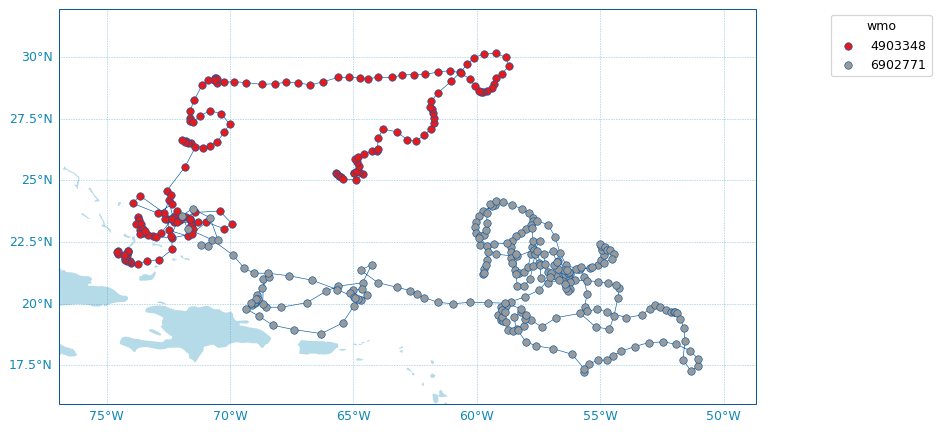Arguments can be passed explicitly as well:

```scatter_map(df,
x='longitude',
y='latitude',
hue='wmo',
cmap='Set1',
traj_axis='wmo')
```

Some options are available to customise the plot, for instance:

```fig, ax = scatter_map(df,
figsize=(10,6),
set_global=True,
markersize=2,
markeredgecolor=None,
legend_title='Floats WMO',
cmap='Set2')
```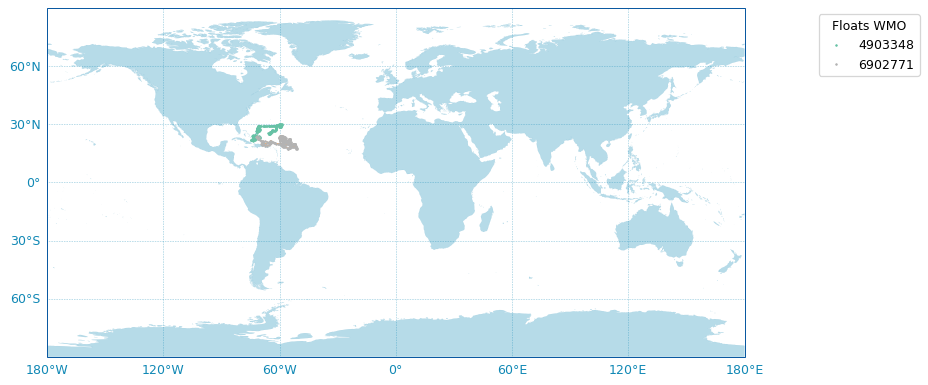### Use predefined Argo Colors#

The `argopy.plot.scatter_map` function uses the `argopy.plot.ArgoColors` utility class to better resolve discrete colormaps of known variables. The colormap is automatically guessed using the `hue` argument. Here are some examples.

Using guess mode for arguments:

```scatter_map(ds, hue='DATA_MODE')
```

or more explicitly:

```scatter_map(ds,
x='LONGITUDE',
y='LATITUDE',
hue='DATA_MODE',
cmap='data_mode',
traj_axis='PLATFORM_NUMBER')
```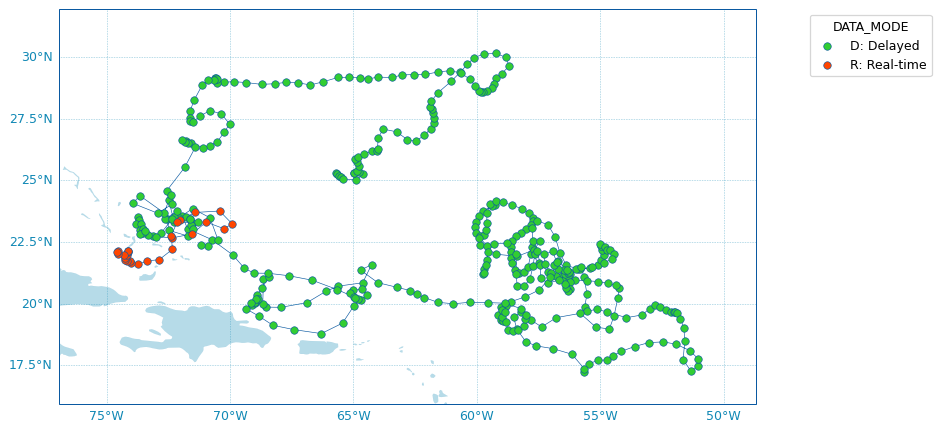### Use any colormap#

Beyond the predefined set of Argo colors, one can use any colormap that can be discretesized.

In the example below, we plot profile years of sampling using the reverse `Spectral` colormap:

```ds['year'] = ds['TIME.year']  # Add new variable to the dataset
scatter_map(ds.isel(N_LEVELS=0),
hue='year',
cmap='Spectral_r',
legend_title='Year of sampling')
```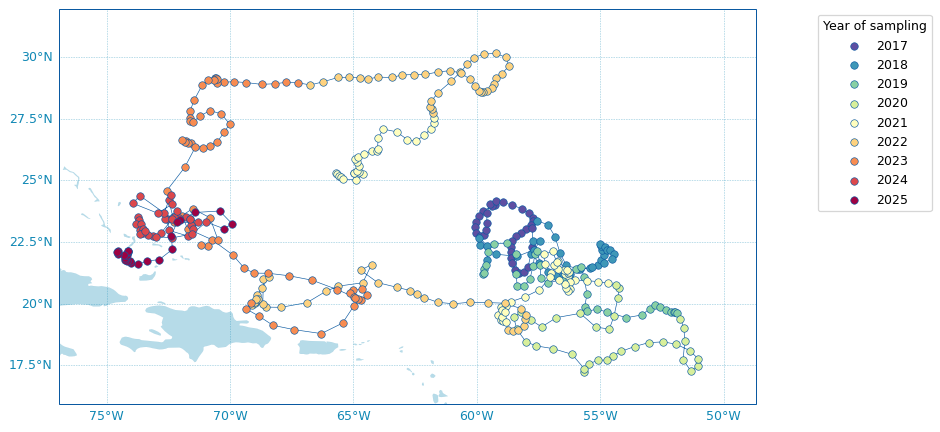## Argo colors#

For your own plot methods, argopy provides the `argopy.plot.ArgoColors` utility class to better resolve discrete colormaps of known Argo variables.

The class `argopy.plot.ArgoColors` is used to get a discrete colormap, as a `matplotlib.colors.LinearSegmentedColormap`.

The Use predefined Argo Colors section above gives examples of the available colormaps that are also summarized here:

```ArgoColors('data_mode')
```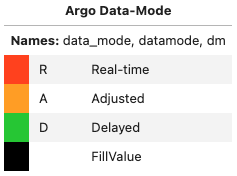```In : ArgoColors('data_mode').definition
Out:
{'name': 'Argo Data-Mode',
'aka': ['datamode', 'dm'],
'constructor': <bound method ArgoColors._colormap_datamode of <argopy.plot.argo_colors.ArgoColors object at 0x7f0755bbd8b0>>,
'ticks': ['R', 'A', 'D'],
Note that `argopy.plot.ArgoColors` can also be used to discretise any colormap:
```ArgoColors('Blues')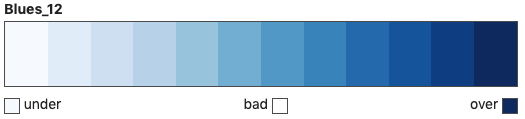```ArgoColors('bwr', N=13)